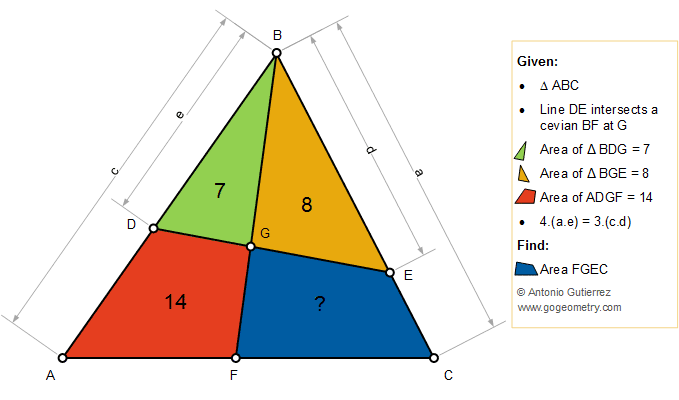# Geometry Problem 1422: Triangle, Transversal, Cevian, Area, Quadrilateral,  Measurement

The figure below shows a triangle ABC. Line DE intersects a cevian BF at G. If area of triangle BDG = 7, area of triangle BGE = 8, area of quadrilateral ADGF = 14, and 4.(BC.BD) = 3.(AB.BE), find the area of quadrilateral FGEC.### Geometric Art of Problem 1422: Sketching, Typography, iPad Apps